```REFERENCE SECTION: CHEMICAL EQUATIONS (1)
Chemical equations represent an essential part of the common language
of scientists. Accordingly, one needs to acquire the skills in both
their construction and interpretation. Setting aside the important fact
that all equations are derived from experiments, their construction
'simply' requires practice and patience using, wherever possible, model
examples: so, the text below focuses on the provision of a sound basis
for their interpretation.
A Chemical Equation
In a chemical reaction, the starting materials are known as reactants
and the substances formed as products. A chemical equation is used to
partially summarize a reaction. Thus, using chemical symbols, reactants
are placed on the left and products on the right of an arrow; and, to
conform with the Law of the Conservation of Mass, there must be the
same number of each type of atom on both sides of the equation; e.g.,```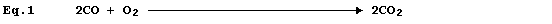```Equation 1, which summarizes the combustion of carbon monoxide, has two
equivalent meanings: first, 'two molecules of gaseous carbon monoxide
and one molecule of gaseous dioxygen react to give two molecules of
gaseous carbon dioxide'; and second, 'two moles of gaseous carbon
monoxide and one mole of gaseous dioxygen react to give two moles of
gaseous carbon dioxide'.
Equation 1 is absolutely complete, in the sense that nothing needs to
be added. Nevertheless, in attempts to provide more complete summaries
of reactions, scientists often festoon equations with a wide variety of
additional information; furthermore, equations are often replaced by
reaction schemes ...
State Symbols
Such symbols are abbreviations for the physical states of the reactants
and products; (aq), (g), (l), and (s) denote 'dissolved in water', gas,
liquid, and solid, respectively. In the absence of state symbols, the
reactants and products are assumed to be in their usual physical states
at room temperature (288 - 298 K) and pressure (100 kPa).
Equation 2 is merely equation 1 modified to include state symbols; and,
in this particular example, cannot be regarded as a useful improvement.```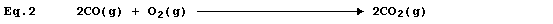```However, the absence of state symbols can result in errors. *
Equation 3 has adorned many textbooks, examination papers, and scripts.```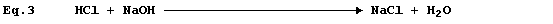```The precise interpretation of Equation 3 is, 'one mole of gaseous
hydrogen chloride and one mole of solid sodium hydroxide react to give
one mole of solid sodium chloride and one mole of liquid water': but,
under rigorously anhydrous conditions, such a chemical reaction would
be unlikely to occur; i.e.,```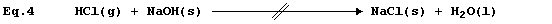```Equation 3 is frequently used to summarise the neutralization reaction
between aqueous solutions of hydrochloric acid and sodium hydroxide:
but the correct equation for this reaction is as follows.```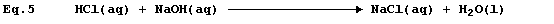```*  The following anecdote may be illuminating. If there is one 'fact'
that people of un certain âge remember, from their best-forgotten days
studying Chemistry, it is that copper(II) sulfate is blue ...``````Energy Changes
Each chemical reaction is accompanied by the formation of one or more
new substances and an energy change (DE). Various symbols are used to
provide a qualitative (or quantitative) indication of such a change in
either a single reaction or a process, in particular: *
- DE, exergonic (i.e., energy is released to the surroundings);
+ DE, endergonic (i.e., energy is absorbed from the surroundings);
- DH, exothermic (i.e., heat energy is released to the surroundings);
+ DH, endothermic (i.e., heat energy is absorbed from the surroundings).
Reaction Conditions
Most chemical reactions are carried out under ambient conditions; i.e.,
under laboratory lighting at room temperature and pressure. Information
about reaction conditions, other than ambient, is placed on top of the
arrow; e.g., named catalyst, light, heat, low or high temperature, and
low or high pressure.
Reversible Reactions
In principle, each chemical reaction is reversible; i.e., it can occur
in both directions (and can be made to proceed in either direction by
altering the conditions): and so each equation should contain a double-
headed arrow. In practice, however, conditions are chosen which usually
ensure that there is complete conversion of reactant(s) to products(s);
and if this has been shown (or assumed) to be so, then such a reaction
is considered to be irreversible and is correctly summarized by an
equation which contains a single-headed arrow.
In industry, it is not always feasible to use conditions which ensure
irreversibility; thus, primarily for reasons of safety and economics,
many industrial-scale syntheses involve reversible reactions; e.g., ...
Nitrogen monoxide, an intermediate in the manufacture of nitric acid,
is obtained by the catalytic oxidation of ammonia; the symbol equation
for the industrial-scale synthesis of this gas is:```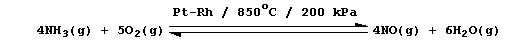```The meaning of this equation is as follows: 'at a temperature of 850°C
and a pressure of 200 kPa, in the presence of a platinum-rhodium
co-catalyst, the reaction of gaseous ammonia and dioxygen is reversible
(with incomplete conversion to gaseous nitrogen monoxide and water); in
a closed system, the forward and reverse reaction rates will be equal
at dynamic equilibrium'.
Reaction Schemes
A chemical equation is, strictly speaking, the only correct method of
summarizing a reaction. Nevertheless, in the scientific literature, the
appearances of such equations are fairly rare. One reason for their
rarity is that many symbol equations are quite complicated; e.g., the
oxidation of ethanol to ethanoic acid by acidified dichromate(VI) is:``````So, rather than bespatter their text with such 'monstrosities', authors
prefer to use a reaction scheme. In this 'chemical shorthand', only the
reactant and product of interest are stated explicitly; the reagent(s)
and conditions are placed over the arrow. So, the oxidation of ethanol
to ethanoic acid, for example, might be summarized by this scheme.```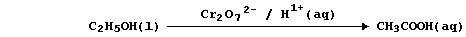```*  In more advanced studies, the commonest symbol used to indicate the
energy change of a reaction is DG (i.e., the change in 'free energy').
Dr. R. Peters                   Next                     Contents' List```